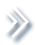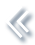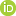####ArchivesReview Articles

## Bayesian quantile semiparametric mixed-effects double regression models

Duo Zhang ,

Department of Mathematical Sciences, Michigan Technological University, Houghton, MI, USALiucang Wu ,

Faculty of Science, Kunming University of Science and Technology, Kunming, People’s Republic of ChinaKeying Ye ,

Department of Management Science and Statistics, The University of Texas at San Antonio, San Antonio, TX, USAMin Wang

Department of Management Science and Statistics, The University of Texas at San Antonio, San Antonio, TX, USAPages 303–315 | Received 07 Feb. 2020, Accepted 15 Jan. 2021, Published online: 05 Feb. 2021,
• Abstract
• References
• Citations

Semiparametric mixed-effects double regression models have been used for analysis of longitudinal data in a variety of applications, as they allow researchers to jointly model the mean and variance of the mixed-effects as a function of predictors. However, these models are commonly estimated based on the normality assumption for the errors and the results may thus be sensitive to outliers and/or heavy-tailed data. Quantile regression is an ideal alternative to deal with these problems, as it is insensitive to heteroscedasticity and outliers and can make statistical analysis more robust. In this paper, we consider Bayesian quantile regression analysis for semiparametric mixed-effects double regression models based on the asymmetric Laplace distribution for the errors. We construct a Bayesian hierarchical model and then develop an efficient Markov chain Monte Carlo sampling algorithm to generate posterior samples from the full posterior distributions to conduct the posterior inference. The performance of the proposed procedure is evaluated through simulation studies and a real data application.

## References

• errors. Journal of Econometrics, 64, 183206. https://doi.org/10.1016/0304-4076(94)90063-9
• Fan, J., & Gijbels, I. (1996). Local polynomial modelling and its applications. In Monographs on statistics and applied probability, vol. 66. Chapman & Hall. [Google Scholar]
• Fan, J., & Li, R. (2004). New estimation and model selection procedures for semiparametric modeling in longitudinal data analysis. Journal of the American Statistical Association, 99, 710723. https://doi.org/10.1198/016214504000001060
• Fonseca, T., Ferreira, Marco A., & Migon, H. (2008). Objective Bayesian analysis for the student-t regression model. Biometrika, 95, 325333. https://doi.org/10.1093/biomet/asn001
• Gelman, A. R., & Gilks, W. (1996). Efficient metropolis jumping rules. In Bayesian statistics, 5 (Alicante, 1994), (pp. 599–607). Oxford Sci. Publ. Oxford University Press. [Google Scholar]
• Geraci, M. (2019). Additive quantile regression for clustered data with an application to children's physical activity. Journal of the Royal Statistical Society. Series C, Applied Statistics, 68, 10711089. https://doi.org/10.1111/rssc.2019.68.issue-4
• Geraci, M., & Bottai, M. (2014). Linear quantile mixed models. Statistics and Computing, 24, 461479. https://doi.org/10.1007/s11222-013-9381-9
• Geweke, J. (1992). Evaluating the accuracy of sampling-based approaches to the calculation of posterior moments. In Bayesian statistics, 4 (Peñíscola, 1991) (pp. 169–193). Oxford University Press. [Google Scholar]
• He, X., Fung, W. K., & Zhu, Z. (2005). Robust estimation in generalized partial linear models for clustered data. Journal of the American Statistical Association, 100, 11761184. https://doi.org/10.1198/016214505000000277
• Hunter, D., & Lange, K. (2000). Quantile regression via an MM algorithm. Journal of Computational and Graphical Statistics, 9, 6077. https://doi.org/10.2307/1390613
• Kai, B., Li, R., & Zou, H. (2011). New efficient estimation and variable selection methods for semiparametric varying-coefficient partially linear models. The Annals of Statistics, 39, 305332. https://doi.org/10.1214/10-AOS842
• Kang, S., Liu, G., Qi, H., & Wang, M. (2018). Bayesian variance changepoint detection in linear models with symmetric heavy-tailed errors. Computational Economics, 52, 459477. https://doi.org/10.1007/s10614-017-9690-8
• Koenker, R., & Bassett, Jr. G. (1978). Regression quantiles. Econometrica, 46, 3350. https://doi.org/10.2307/1913643
• Koenker, R., Chernozhukov, V., He, X., & Peng, L. (2018). Handbook of quantile regression. In Chapman & Hall/CRC handbooks of modern statistical methods. CRC Press. [Google Scholar]
• Koenker, R., & Park, B. (1996). An interior point algorithm for nonlinear quantile regression. Journal of Econometrics, 71, 265283. https://doi.org/10.1016/0304-4076(96)84507-6
• Kotz, S., Kozubowski, T. J., & Podgórski, K. (2001). The laplace distribution and generalizations: A revisit with applications to communications, economics, engineering, and finance. Birkhäuser Boston, Inc[Crossref]
• Kozumi, H., & Kobayashi, G. (2011). Gibbs sampling methods for Bayesian quantile regression. Journal of Statistical Computation and Simulation, 81, 15651578. https://doi.org/10.1080/00949655.2010.496117
• Lombardia, M. J., & Sperlich, S. (2007). Semiparametric inference in generalized mixed effects models. Journal of the Royal Statistical Society. Series B. Statistical Methodology, 70, 913930. https://doi.org/10.1111/j.1467-9868.2008.00655.x
• Raftery, A., & Lewis, S. (1992). [Practical Markov Chain Monte Carlo]: comment: one long run with diagnostics: implementation strategies for Markov Chain Monte Carlo. Statistical Science, 7, 493497. https://doi.org/10.1214/ss/1177011143 [Crossref]
• Scheike, T. H., & Zhang, M. -J. (2011). Analyzing competing risk data using the R timereg package. Journal of Statistical Software, 38, 115. https://doi.org/10.18637/jss.v038.i02
• Tang, N., & Duan, X. (2012). A semiparametric Bayesian approach to generalized partial linear mixed models for longitudinal data. Computational Statistics & Data Analysis, 56, 43484365. https://doi.org/10.1016/j.csda.2012.03.018
• Tian, Y., Li, E., & Tian, M. (2016). Bayesian joint quantile regression for mixed effects models with censoring and errors in covariates. Computational Statistics, 31, 10311057. https://doi.org/10.1007/s00180-016-0659-1
• Tian, Y., & Song, X. (2020). Bayesian bridge-randomized penalized quantile regression. Computational Statistics & Data Analysis, 144, 106876, 16. https://doi.org/10.1016/j.csda.2019.106876
• Tian, Y., Tian, M., & Zhu, Q. (2014). Linear quantile regression based on EM algorithm. Communication in Statistics – Theory and Methods, 43, 34643484. https://doi.org/10.1080/03610926.2013.766339
• Waldmann, E., Kneib, T., Yue, Y. R., Lang, S., & Flexeder, C. (2013). Bayesian semiparametric additive quantile regression. Statistical Modelling, 13, 223252. https://doi.org/10.1177/1471082X13480650
• Wu, L., Tian, G.-L., Zhang, Y.-Q., & Ma, T. (2017). Variable selection in joint location, scale and skewness models with a skew-t-normal distribution. Statistics and Its Interface, 10, 217227. https://doi.org/10.4310/SII.2017.v10.n2.a6
• Xu, D., Zhang, Z., & Wu, L. (2016). Bayesian analysis for semiparametric mixed-effects double regression models. Hacettepe Journal of Mathematics and Statistics, 41, 279296[Google Scholar]
• Yang, Y., Wang, H. J., & He, X. (2016). Posterior inference in Bayesian quantile regression with asymmetric Laplace likelihood. International Statistical Review, 84, 327344. https://doi.org/10.1111/insr.12114
• Yu, K., & Moyeed, R. A. (2001). Bayesian quantile regression. Statistics & Probability Letters, 54, 437447. https://doi.org/10.1016/S0167-7152(01)00124-9
• Zhang, H., Huang, Y., Wang, W., Chen, H., & Langland-Orban, B. (2019). Bayesian quantile regression-based partially linear mixed-effects joint models for longitudinal data with multiple features. Statistical Methods in Medical Research, 28, 569588. https://doi.org/10.1177/0962280217730852
• Zhao, P., & Xue, L. (2010). Variable selection for semiparametric varying coefficient partially linear errors-in-variables models. Journal of Multivariate Analysis, 101, 18721883. https://doi.org/10.1016/j.jmva.2010.03.005
• Zhao, P., & Xue, L. (2011). Variable selection for semiparametric varying-coefficient partially linear models with missing response at random. Acta Mathematica Sinica, English Series, 27, 22052216. https://doi.org/10.1007/s10114-011-9200-1

To cite this article: Duo Zhang, Liucang Wu, Keying Ye & Min Wang (2021) Bayesian quantile
semiparametric mixed-effects double regression models, Statistical Theory and Related Fields, 5:4,303-315, DOI: 10.1080/24754269.2021.1877961